# Resolver Decoder

Compute motor mechanical position and speed as well as sine and cosine values of motor electrical position

• Library:
• Motor Control Blockset / Sensor Decoders

•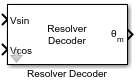## Description

The Resolver Decoder block computes the following for a resolver connected to a motor shaft:

• Mechanical angular position of motor

• Sine and cosine of electrical angular position of motor

• Mechanical speed of motor

### Resolver Operation

A resolver uses a primary excitation input signal to generate the modulated secondary sine and cosine waveforms, which is then sampled by the ADC. The resolver utilizes one winding to two winding transformations. The sine and cosine modulation occurs in the secondary windings because of the design and construction of these windings, which places them at positions that are 90 degrees apart.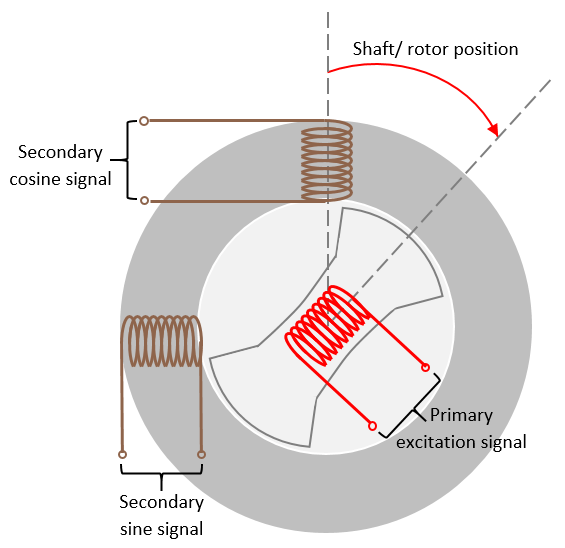### Block algorithm

The block uses the correctly sampled and normalized version of secondary sine and cosine waveforms to demodulate the sine and cosine signals using which it determines the electrical position of the resolver. It converts this position into its mechanical equivalent according to the number of pole pairs available in the resolver. The resulting value indicates the mechanical position of the motor.

The block also uses the demodulated sine and cosine signals (sine and cosine of resolver electrical angle) to compute the motor speed as well as the sine and cosine values of the motor electrical position.

### Resolver excitation methods

The block supports the following two excitation methods:

• Sinusoidal excitation — When the primary excitation signal is sinusoidal. This generates sinusoidal secondary waveforms after modulation.

• Square pulse excitation — When the primary excitation signal is a square pulse signal. This generates square pulse secondary signals after modulation.

### Sinusoidal excitation

When you use sinusoidal excitation method, the block expects the secondary sine and cosine resolver signals that are sampled, by default, at the rate of 16 samples per excitation signal cycle, as shown below. It may also add a phase delay to the sampled sine and cosine signals with respect to the excitation signal.

When using sinusoidal excitation, the block does not normalize the modulated sine and cosine resolver output automatically. Normalize these modulated waveforms (within the range of [-1,1] and centred at 0) before you provide them to the block.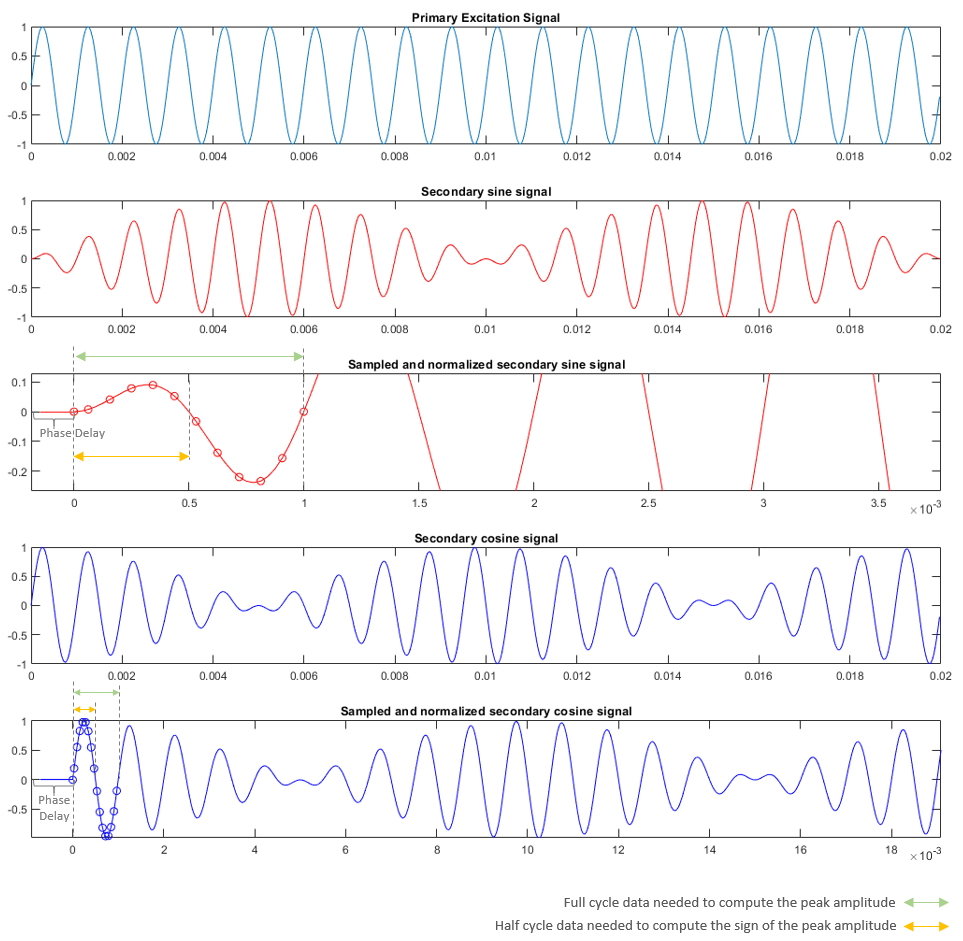The block then demodulates the sampled signal. It computes the average, peak amplitude values, and the sign of the peak amplitude of a signal cycle as:

The block computes the average, peak amplitude values, and the sign of the peak amplitude of a signal cycle as

`${Å}_{average}=\frac{1}{n}\sum _{i=0}^{n-1}\left(|{Å}_{i}|\right)$`
`${Å}_{peak}={Å}_{average}×\frac{\pi }{2}$`

where:

• ${Å}_{average}$ is the average amplitude value of a signal cycle

• $n$ is the number of samples per excitation cycle

• ${Å}_{peak}$ is the peak amplitude value of a signal cycle

The block computes the electrical angular position of the resolver as

where:

• ${u}_{\text{sin}_peak}$ is the ${Å}_{peak}$ of the secondary sine signal

• ${u}_{\text{cos}_peak}$ is the ${Å}_{peak}$ of the secondary cosine signal

• $\theta$ is the electrical angular position of the resolver

This enables the block to demodulate and extract the sine and cosine envelopes. It uses these demodulated envelop signals to compute the block outputs.

### Square pulse excitation

When using the square pulse excitation method, the block expects the secondary sine and cosine signals sampled at the rate of one sample every pulse as shown below.

If you select Enable input normalization field, then after receiving the discrete time sampled waveform, the block automatically normalizes the waveform (within the range of [-1,1] and centred at 0). To perform normalization within the block, ensure that both input signals have equal peak magnitudes.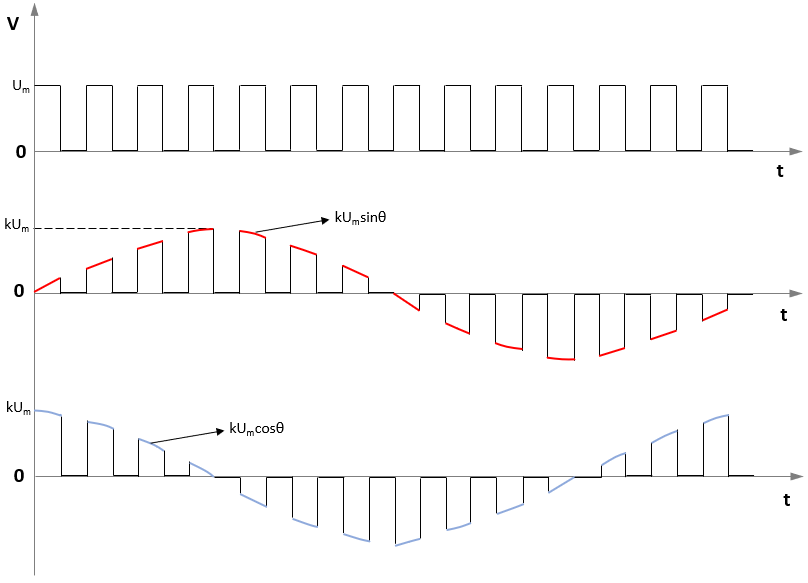It demodulates the sine and cosine signals. It uses these demodulated signals to compute the block outputs.

### Computation of motor mechanical position

When using either sinusoidal or square pulse excitation, the block uses the demodulated waveforms to compute the electrical position of the resolver. This resolver position may vary using either a positive ramp (for clockwise rotation) or a negative ramp (for anti-clockwise rotation).

To correctly detect the wrap-around (from 0 PU to 1 PU or from 1 PU to 0 PU), the block measures the difference between the two consecutive samples. Because the input signal frequency is always less than ½ of sampling frequency, a difference less than -0.5 PU indicates a positive ramp whereas a difference that is less than +0.5 PU indicates a negative ramp.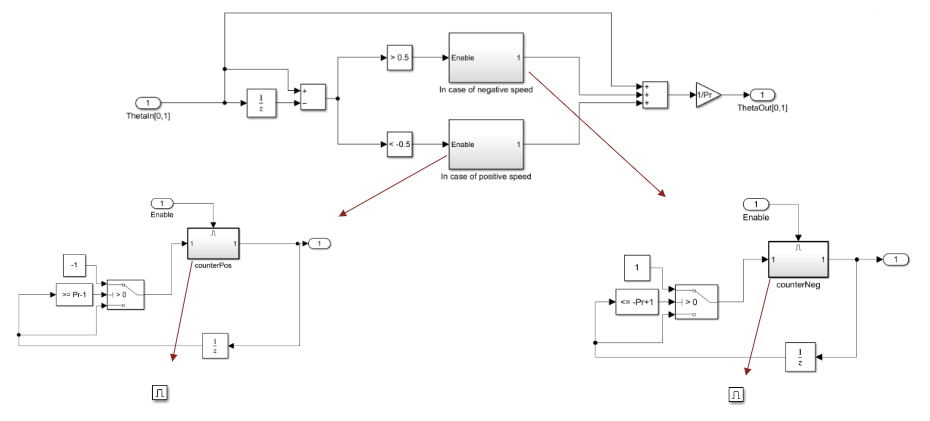After identifying the resolver electrical position direction, the block uses the number of resolver pole pairs to compute the mechanical position of the resolver (and the motor) by extrapolating the ramp signal.

For example, for a 3-pole pair resolver, the block extrapolates the ramp to achieve a magnitude that is 3 times the original magnitude. It then performs a division by 3 to obtain the resolver mechanical position as shown below.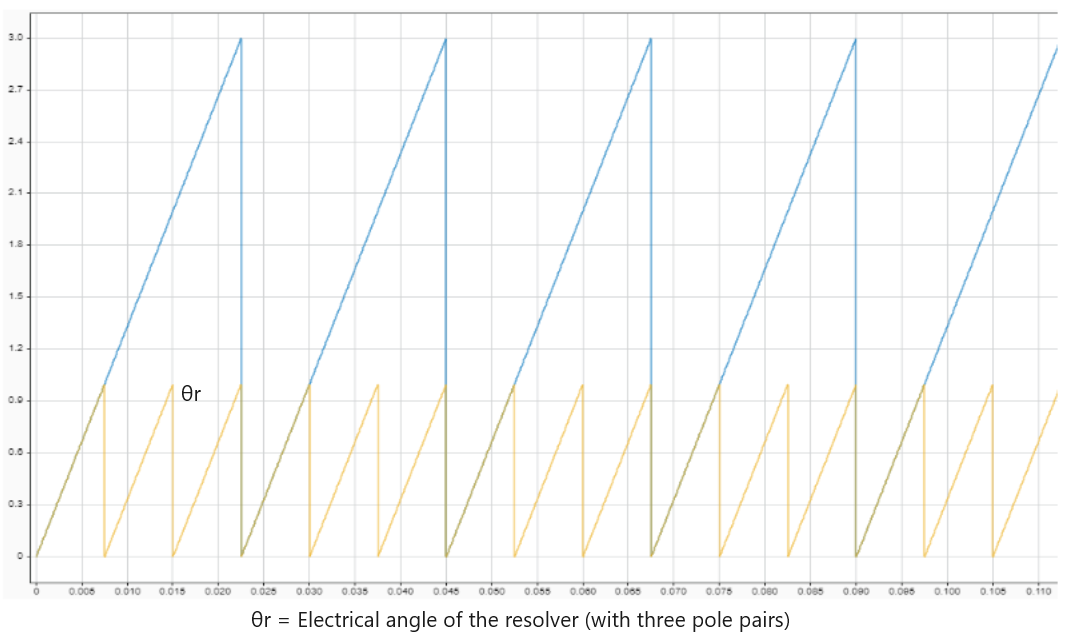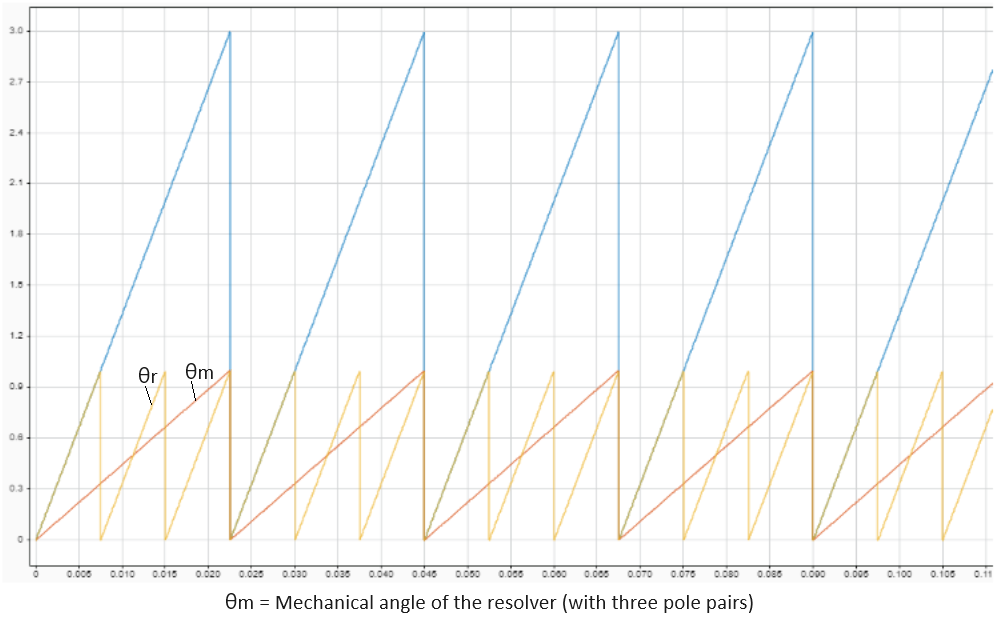### Computation of sine and cosine of electrical motor position

When using either sinusoidal or square pulse excitation, the block generates the demodulated sine and cosine waveforms (sine and cosine of resolver electrical position). From these demodulated waveforms, the block computes sine and cosine of motor electrical position using an arithmetic computation according to the ratio of motor and resolver pole pairs. To accommodate different possible ratios, the block utilizes unit blocks, which in turn use a generic binary-coded decimal (BCD) based structure.

For example, if the ratio of motor and resolver pole pairs (n) is 5, the following image indicates the BCD based algorithm that eventually generates sine and cosine of motor electrical position.

Therefore, n = number of motor pole pairs/ number of resolver pole pairs = 5.

BCD code for n = `5` is ```[0 0 1 0 1]``` or ```[24 23 22 21 20]```. The block configures the algorithm for this sequence as shown below.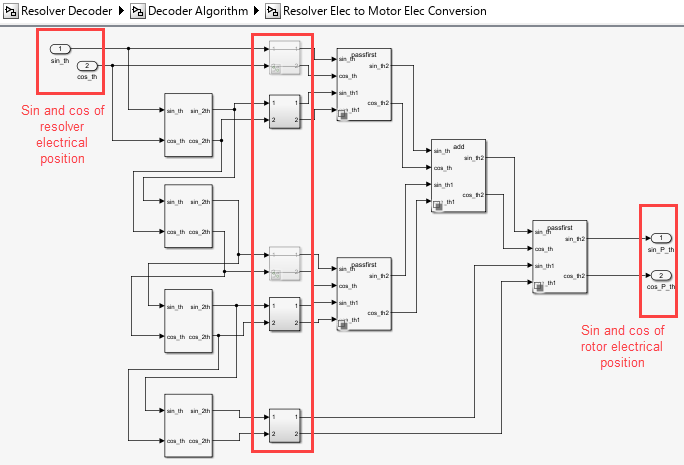The block supports values of n (integers) ranging from 1 to 31.

Note

The block optimizes the computation according to the ratio n. For example, if n = `5`, the block only computes `sin_2th` and `cos_2th` twice by utilizing the following two subsystems only.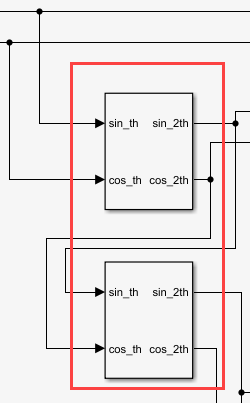Similarly, whenever a switch does not bypass the signals, it terminates them as shown below to ensure minimal and optimized code generation.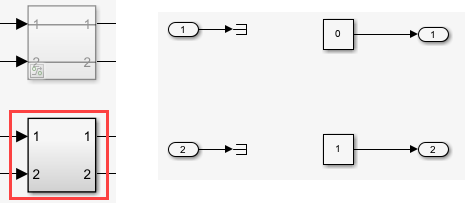### Computation of motor speed

When using either sinusoidal or square pulse excitation, the block generates the demodulated sine and cosine waveforms (sine and cosine of resolver electrical position). From these waveforms, the block computes the motor mechanical speed using the algorithm shown below.

At first, it computes the difference between the two samples of these discrete time sampled signals and adds them:

In the ideal scenario, when using continuous time signals:

Where, ωr is constant with respect to time.

Because we use discrete time sampled signals:`$\mathrm{cos}\theta \frac{\Delta \mathrm{sin}\theta }{\Delta t}-\mathrm{sin}\theta \frac{\Delta \mathrm{cos}\theta }{\Delta t}$`

`$\frac{\Delta \mathrm{sin}\theta }{\Delta t}=\frac{\mathrm{sin}{\omega }_{r}{T}_{s}n-\mathrm{sin}\left[{\omega }_{r}{T}_{s}\left(n-1\right)\right]}{{T}_{s}}$`
`$\frac{\Delta \mathrm{cos}\theta }{\Delta t}=\frac{\mathrm{cos}{\omega }_{r}{T}_{s}n-\mathrm{cos}\left[{\omega }_{r}{T}_{s}\left(n-1\right)\right]}{{T}_{s}}$`
`$\mathrm{cos}\theta \frac{\Delta \mathrm{sin}\theta }{\Delta t}-\mathrm{sin}\theta \frac{\Delta \mathrm{cos}\theta }{\Delta t}=\frac{\mathrm{sin}{\omega }_{r}{T}_{s}}{{T}_{s}}$`
`$\Delta t={T}_{s}$`
`$\frac{\mathrm{cos}\theta \cdot \Delta \mathrm{sin}\theta }{{T}_{s}}-\frac{\mathrm{sin}\theta \cdot \Delta \mathrm{cos}\theta }{{T}_{s}}=\frac{\mathrm{sin}{\omega }_{r}{T}_{s}}{{T}_{s}}$`
`$\mathrm{cos}\theta \cdot \Delta \mathrm{sin}\theta -\mathrm{sin}\theta \cdot \Delta \mathrm{cos}\theta =\mathrm{sin}{\omega }_{r}{T}_{s}$`

If ωxTs is very small, then sinωrTs approximately equals to ωrTs. This is `speed x time`, therefore, this term indicates position difference for a sample duration `Δθ`.

`$\mathrm{cos}\theta \cdot \Delta \mathrm{sin}\theta -\mathrm{sin}\theta \cdot \Delta \mathrm{cos}\theta =\Delta \theta =\mathrm{sin}{\omega }_{r}{T}_{s}\approx {\omega }_{r}{T}_{s}$`
`${\omega }_{r}=\frac{1}{{T}_{s}}{\mathrm{sin}}^{-1}\Delta \theta$`
`${\omega }_{r}\approx \frac{\Delta \theta }{{T}_{s}}$`

To obtain a more accurate speed, we use the Taylor series expansion of sin-1 to add the following compensation of $\frac{\Delta {\theta }^{3}}{6}$.

`${\omega }_{r}=\frac{1}{{T}_{s}}{\mathrm{sin}}^{-1}\Delta \theta$`
`$⇒{\omega }_{r}\approx \frac{1}{{T}_{s}}\left[\Delta \theta +\frac{\Delta {\theta }^{3}}{6}\right]$`

Because the error is negligible, the preceding ωr value can be considered accurate. Therefore, we can state:

`${\omega }_{r}=\frac{1}{{T}_{s}}\left[\Delta \theta +\frac{\Delta {\theta }^{3}}{6}\right]$`
`${\omega }_{r}=\left(\frac{g}{{T}_{s}}\right)\left[\Delta \theta +\frac{\Delta {\theta }^{3}}{6}\right]$`

`$\omega =\left(\frac{g}{{T}_{s}Pr}\right)\left[\Delta \theta +\frac{\Delta {\theta }^{3}}{6}\right]$`

Where, Pr is the number of pole pairs of resolver.

The block multiplies the gain of $\left(\frac{g}{{T}_{s}Pr}\right)$ separately to avoid data overflow when using fixed point data type.

Here, $\frac{\Delta {\theta }^{3}}{6}$ is the approximate compensation that the block applies to the computed speed as shown below to obtain more accurate version of the actual mechanical speed.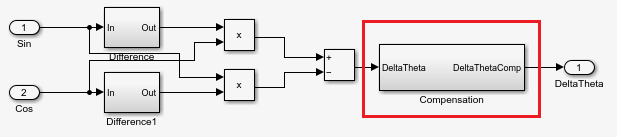Where:

• g is the speed conversion factor.

• θ is the electrical position of the resolver.

• ωr is the electrical speed of the resolver.

• Δθ is the resolver electrical position difference per sample.

• ω is the mechanical speed of the resolver (or motor).

• Ts is the sampling time of the sine and cosine envelop signals.

Note

The block inputs should have identical amplitude and data types (either signed fixed or floating point).

## Ports

### Input

expand all

Secondary sine waveform output from the resolver that is sampled. When using sinusoidal excitation, this signal should be normalized within the range of [-1, 1] and centred at 0.

Data Types: `single` | `double` | `fixed point`

Secondary cosine waveform output from the resolver that is sampled. When using sinusoidal excitation, this signal should be normalized within the range of [-1, 1] and centred at 0.

Data Types: `single` | `double` | `fixed point`

### Output

expand all

Mechanical angular position of the resolver (and the motor) in either degrees, radians, or per-unit.

Data Types: `single` | `double` | `fixed point`

Sine of electrical angular position of the motor.

Data Types: `single` | `double` | `fixed point`

Cosine of electrical angular position of the motor.

Data Types: `single` | `double` | `fixed point`

Mechanical speed of the motor in either degrees/sec, radians/sec, RPM, or per-unit.

Data Types: `single` | `double` | `fixed point`

## Parameters

expand all

The output of the Resolver Decoder block:

• Mechanical position — Enable the block to compute motor mechanical position.

• Sine and Cosine electrical position — Enable the block to compute sine and cosine values of motor electrical position.

• Mechanical speed — Enable the block to compute the mechanical speed of the motor.

### Input Parameters

The number of pole pairs available in the resolver.

The resolver excitation method used to obtain secondary sine and cosine waveforms.

When using square pulse excitation method, the block normalizes the secondary sine and cosine inputs only if you select this parameter.

#### Dependencies

To enable this parameter, set Type of excitation method to `Square Pulse Excitation`.

### Inputs for sinusoidal excitation method

The phase delay that the block must add to the `Sin` and `Cos` input port signals.

#### Dependencies

To enable this parameter, set Type of excitation method to `Sinusoidal Excitation`.

Number of samples available in one cycle of the `Sin` and `Cos` input port signals.

#### Dependencies

To enable this parameter, set Type of excitation method to `Sinusoidal Excitation`.

### Inputs for Sine and Cosine electrical position

The number of pole pairs available in the motor.

#### Dependencies

To enable this parameter, set Block Output to `Sine and Cosine electrical position`.

The offset between resolver mechanical position and motor mechanical position (in radians).

#### Dependencies

To enable this parameter, set Block Output to `Sine and Cosine electrical position`.

Select the rounding mode for fixed-point operations used when computing sine and cosine of motor electrical position. You can select:

• Simplest — Generates compact rounding code with faster computation. However, with time, it may add bias in sine and cosine electrical position output.

• Zero — Ensures that the rounding code does not add any bias in the sine and cosine electrical position output. However, this results in slower computation.

#### Dependencies

To enable this parameter, set Block Output to `Sine and Cosine electrical position`.

The fixed time interval (in seconds) between every two consecutive instances of block execution.

#### Dependencies

To enable this parameter, set Block Output to `Mechanical speed`.

### Output Parameters

The unit of the mechanical position output of the block.

#### Dependencies

To enable this parameter, set Block Output to `Mechanical position`.

The unit of the mechanical speed output of the block.

#### Dependencies

To enable this parameter, set Block Output to `Mechanical speed`.

The rated speed of the motor (in RPM).

#### Dependencies

To enable this parameter, set Speed unit to `Per-Unit` and Block Output to `Mechanical speed`.

## Version History

Introduced in R2020a